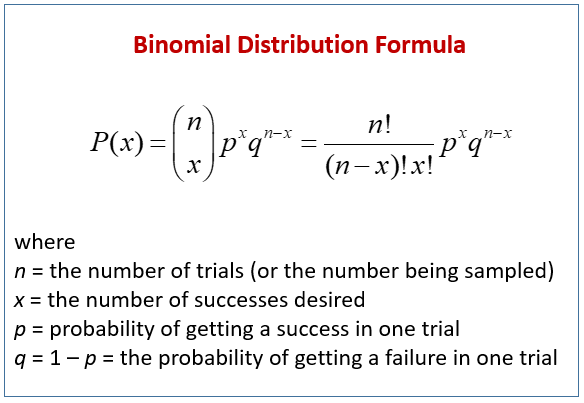There are two basic categories of data: discrete and continuous. Continuous data is measurable and can take on an almost infinite number of values such as time, weight or volume. Discrete data is countable and can be described as counts or categories such as defects or defectives.

## Overview: What is a binomial random variable?

The Merriam-Webster dictionary defines the word bi as follows:

a: two – bilateral

b: coming or occurring every two – bicentennial

c: into two parts – bisect

d: coming or occurring two times – biannual

Therefore, we can conclude the word binomial refers to something that has two outcomes or conditions. We can define a binomial random variable as a count of things which have only two possible outcomes which occur in a fixed number of trials. For example, you can count the number of defective parts out of 100 units produced. The part can only be defined having one of two possible outcomes: defective or not defective

For a variable to be a binomial random variable, the following conditions must be met:

• There are a fixed number of trials or a constant sample size – flip a coin 50 times
• There are only two possible outcomes for each trial – you can only get a head or tail
• The probability of occurrence is the same on each trial – 50/50 chance of a head or tail
• Trials are independent of each other – each flip of the coin is independent

If you plot all the possible probabilities, you can create a distribution called the binomial distribution. The binomial distribution summarizes the probability of observing a specific number of binary outcomes in a specific number of trials. The formula is:Binomial Distribution

## An industry example of a binomial random variable

The head of engineering wanted to estimate the probability of producing a certain number of defectives on the next production run. Historically, the process produced about a 3.5% defective rate. A run is usually about 1,000 pieces.

The customer who will be receiving these parts has a stated expectation of receiving no more than 2.5% defective. The head of engineering wanted to know the probability of meeting that expectation.

He chose to use the formula for a binomial distribution with his n=1,000, x= 25 (2.5% of 1,000), p=.035 and q=.965. He came up with a probability of about 1.56% for meeting his customer’s expectation. Since there was not sufficient time to change the process, he had to temporarily do extensive inspection to separate the defectives before shipping the product.

### Is a binomial random variable discrete or continuous data?

It is discrete since you count the number of occurrences, not measure them.

### Why is it called a binomial random variable?

The word bi means two. In this context it means you have discrete data with only two possible outcomes. For example, if you flip a fair coin, there are only two possible outcomes, heads, or tails for each flip. Your probability is 50/50 for each flip. If you flip it 30 times, you can count the number of heads and tails.

### What if I want to know the probability of getting 30 heads if I flip a fair coin 50 times?

You can use the binomial distribution formula shown below where n=50, x=30, p=.5 and q=.5: